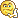www.vustudents.ning.com

We non-commercial site working hard since 2009 to facilitate learning Read More. We can't keep up without your support. Donate.

Question No. 1:
a) You are a bank manager and given the responsibility to manage the liquidity risk being faced by the bank. The Balance Sheet of the bank is given below:
Table: Balance sheet of a bank holding no excess reserves
Assets (in Million)
Liabilities (in Million)
Reserves Rs.15 million
Deposits Rs.90 million Rs.100million
Loans Rs.95 million
Borrowed funds Rs.35 million
Securities Rs.35 million
Bank capital Rs.20 million
A customer demands Rs.5 million cash withdrawals from the bank; what changes in the above Balance Sheet will occur if you decide to manage the liquidity risk through:
a. Selling the securities
b. Reducing the loans
a. Borrowings
b. Attracting deposits
Note: You are required to prepare four different Balance Sheets for each of the above mentioned strategies. (10 marks)
b) Discuss why bankers prefer liability management over asset management in order to mitigate liquidity risk? (5 marks)
Question No. 2:
a) You, as a bank manager, are managing the bank’s assets and liabilities in such a way that interest rate the bank has to pay on its liabilities is 4% while interest rate the bank charges on its various assets is 6%. Suppose 30% of the bank’s assets fall into the category of interest-sensitive while others are not sensitive to the changes in interest rate. Similarly, half of the bank’s liabilities are interest-sensitive while rests of the half are not. What will be the impact on the profitability of the bank if the interest rate rises by 1% on all assets and liabilities of the bank? (10 marks)
b) What will be the impact of increase in interest rate on the profitability of the bank if the bank has more interest-sensitive liabilities than interest-sensitive assets? (5 marks)

Views: 6589

Attachments:

Replies to This Discussion

Nida please go through the answer(2a) provided by Naveed Iqbal it is absolutely correct

part b:

when bank has more interest rate sensitive liabilities than those interest rate assets, an increase in interest rate will be cut into the bank's profits.

as shown in q no.2 part a.

please don't submit as it is,

good luckThnxxx Naveed Iqbal

Question#1 Solution:

Bond Valuation

Part a)

Bond value = c [1 – 1/(1+r)^n / r] + Par/(1+r)^n

Bond value = 90 [1 – 1/(1+0.10)^12 / 0.10 + 1000/(1+0.10)^12

Bond Value = 90 [1 – 0.3186 /0.10] + 1000/3.1384

Bond value = 90 (6.814) + 318.63

Bond value = 613.26 +318.63

Bond Value = 931

Part b)

Bond value = c [1 – 1/(1+r)^n / r] + Par/(1+r)^n

Bond value = 45 [1 – 1/(1+0.05)^24 / 0.05 + 1000/(1+0.05)^24

Bond Value = 45 [1 – 0.3100 /0.05] + 1000/3.2250

Bond value = 45 (13.8) + 310.07

Bond value = 621 +310.

Bond Value = 931

Part a)

P = Div(1+g)/rF +rP – g

D = 1.80

Rce = rF +rP = 12% \

g = 5%

P = 1.80 (1+0.05) / 12% - 5%

P = 1.80 (1.05) / 7%

P = 1.89/0.07

P = 27

Part b)

Div1= Do(1+g)3

Div1= 1.80 (1+0.05)3

Div1= 1.80 (1.1576)

Div1 = 2.083

P0 = D0 x (1 + g)/(R – g)

P0= 2.083 x 1.04/(0.12 – 0.04)

P0= 2.166 / 0.08

P0= 27.075

kia ye thek hy?

ye bond kahan se aagya :p

any one have slo of MGT 411 plz sahre

yar ye kya hai???ye kia haaa??? :(

Samia ap ne kisi aur subject ki assignment yahan post ki hai...its MGT411 grp....NOTE:

Please do make changes; because it is somewhat similar to handouts… otherwise you will be copied…

Just get hint n do by yourself…

Question 01:

a)

Solved here is accurate………..

b)

• Banks do not like to meet their deposit outflows by contracting the asset side of the balance sheet because doing so shrinks the size of the bank
• Banks can use liability management to obtain additional funds by

Means bankers  prefer  liability  management  over  asset  management  in  order  to mitigate liquidity risk because doing so shrinks the size of bank.

Question 02:

a)

The impact of an interest rate increase on bank profits (per \$100 of assets)

Items                                                    Assets                          Liabilities

Interest rate sensitive                             \$30                              \$50

Not interest rate sensitive                      \$70                              \$50

Initial interest rate                                  6%                               4%

(New interest rate on interest rate          7%                               5%

sensitive assets and liabilities)

Revenue from assets

At initial interest rate                 (0.06×\$30)+(0.06×\$70)=\$6.00

After interest rate change         (0.07×\$30)+(0.06×\$70)=\$6.30

Cost of liabilities

At initial interest rate                 (0.04×\$50)+(0.04×\$50)=\$4.00

After interest rate change          (0.05×\$50)+(0.04×\$50)=\$4.50

Profits at initial interest rate:

(\$6.00) – (\$4.00) = \$2.00 per \$100 in assets

Profits after interest rate change:

(\$6.30) – (\$4.50) = \$1.80 per \$100 in assets

b)

Impact of increase in interest rate on the profitability of the bank is that it cut into the bank’s profits if the bank has more interest-sensitive liabilities than interest-sensitive assets. Means it lower down the profit of the bank.

Latest ActivityMani Siddiqui posted a discussion

How To Apply Kamyab Jawan Program Free Courses /Free training

23 minutes agospacedata posted discussions
2 hours ago